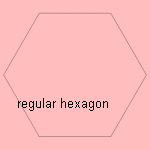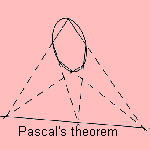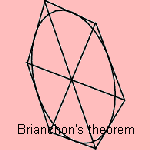# hexagon

## line

last updated: 2005-07-16The regular hexagon can be divided in three regular triangles, so that the figure is easy to construct with a pair of compasses. Already the Greek group of Pythagoras knew that a plane can be filled with regular hexagons.At the age of 16 Pascal proved a theorem (later called after Pascal), for a hexagon inscribed in a conic section: the cuttings of the opposite sides lie on a straight line: the line of Pascal.
The dual of this theorem is Brianchon's theorem: given a hexagon circumscribed on a conic section, the lines joining opposite polygon vertices meet in a single point.
These theorems hold also for irregular and not convex hexagons.

Later on, the hexagon had been given the name of mystical hexagon 1).

In chemistry the regular hexagon is the formula for the benzene ring. It is made of six carbon atoms, bound in a ring by a hydrogen atom between each neighbor. Also some crystal structure have a regular hexagonal form, for instance zinc sulfide and a special form of diamond.

In real life we see the regular hexagon in the ice crystals by which snow is formed 2. Probably Johann Kepler was the first to remark this hexagonal symmetry in snow flakes, and he wrote a book about it for his money-lender (in 1611).
The hexagon form is caused by the three parts of a water molecule: two hydrogen atoms and one oxygen atom.
In construction the hexagonal bolt and the hexagonal screw are used.

In the world of the animals the regular hexagon can be seen in the honey-comb and as the radiate 3).
In 1999 Thomas Hayles proved that the hexagonal form constructed by the honey-bees is the best (most efficient) way to divide a plane in small and equal pieces.
This is related to the fact that the hexagon is the most efficient way to lay circles in a plane. This can be understood by realizing that six equal circles fit precisely around a circle with that same radius.

notes

1) Known as the 'hexagramma mysticum'.

2) All snow flakes have sixfold symmetry, some kinds of snow flakes have the form of a hexagon.

3) An unicellular animal with a silicon skeleton.